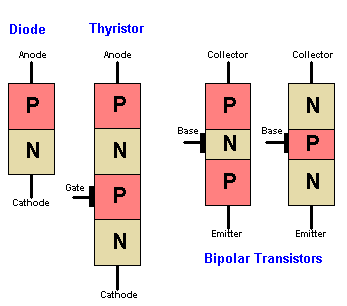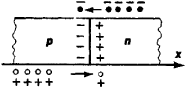# P-N Junction

(redirected from Pn junctions)
Also found in: Dictionary, Thesaurus.
Related to Pn junctions: P-n diode

## PN junction

The interface at which p-type silicon and n-type silicon make contact with each other. At this coupling point, free electrons (n-type) and holes (p-type) cancel each other and form a "depletion zone" that acts as a non-conductive barrier. The PN junction is one of the primary building blocks of semiconductors.

Diodes and Thyristors
A diode (one way valve) is a PN junction with p-type on one side and n-type on the other. When a positive voltage is applied to the p-type side (forward bias), it shrinks and overcomes the depletion zone, causing the current to flow from the p-type to the n-type side. When a negative voltage is applied to the p-type of the diode (reverse bias), it increases the depletion zone and prevents current from flowing. See diode.

Built like two diodes in a row (three PN junctions) but with a third terminal, a thyristor is a one-way valve that, once turned on, lets current flow until it falls below a certain threshold (see thyristor).

Bipolar Transistors
Double PN junctions are used to build bipolar transistors. These p-type/n-type bipolar "sandwiches" are called "PNP" and "NPN" transistors. See bipolar transistor and n-type silicon.

PN JunctionsPlacing p-type silicon next to n-type silicon is the primary way many semiconductor devices are constructed. The field effect transistors (FETs) in CMOS chips also use n-type and p-type silicon, but are designed differently (see FET and MOSFET).
Copyright © 1981-2019 by The Computer Language Company Inc. All Rights reserved. THIS DEFINITION IS FOR PERSONAL USE ONLY. All other reproduction is strictly prohibited without permission from the publisher.
The following article is from The Great Soviet Encyclopedia (1979). It might be outdated or ideologically biased.

## P-N Junction

the region of a crystalline semiconductor in which a spatial change from n-type conductivity to p-type conductivity occurs. In a semiconductor, n-type conductivity is associated with electrons and p-type conductivity is associated with holes.

Because the concentration of holes is much higher in the p-region of a p-n junction than in the p-region, holes from the p-region tend to diffuse into the p-region. Electrons diffuse into the p-region. However, after holes leave the p-region, negatively charged acceptor atoms are left behind; after electrons leave the n-region, positively charged donor atoms are left behind. Since acceptor and donor atoms are stationary, a double space-charge layer called the depletion layer is formed in the region of a p-n junction. The depletion layer consists of negative charges in the p-region and positive charges in the p-region (Figure 1).Figure 1. Diagram of a p-n junction. The solid circles represent electrons; the white circles, holes.

In magnitude and direction, the barrier electric field that arises in the depletion layer is such that it opposes the diffusion of free charge carriers across a p-n junction. Under conditions of thermal equilibrium in the absence of an applied voltage, the total current across a p-n junction is equal to zero. Thus, in a p-n junction, a dynamic equilibrium exists in which a small current produced by minority carriers (electrons in the p-region or holes in the n-region) flows toward the junction and across it under the action of the barrier field and a current that is equal in magnitude and is produced by majority carriers (electrons in the n-region or holes in the p-region) flows across the junction in the opposite direction. In this case, the majority carriers must overcome the potential barrier associated with the barrier field. The potential difference that arises between the p-region and the n-region usually amounts to tenths of a volt; the difference is called the contact potential difference, or the height of the potential barrier.

An applied electric field changes the height of the potential barrier and disturbs the equilibrium of the charge-carrier flows across the barrier. A positive potential applied to the p-region of a p-n junction is referred to as a forward bias. A positive potential applied to the n-region is known as a reverse bias.

If a positive potential is applied to the p-region, the applied field is directed opposite to the barrier field; that is, the potential barrier is lowered. In this case, the number of majority carriers that can overcome the potential barrier increases exponentially as the applied voltage is increased. The concentration of minority carriers on both sides of a p-n junction increases—that is, minority carriers are injected—and, at the same time, equal numbers of majority carriers enter the p-region and the n-region through the terminals. The majority carriers are produced by the charge neutralization of minority carriers. As a result of carrier injection, the rate of electron-hole recombination increases and a nonzero current flows across the junction. When the applied voltage is increased, the current increases exponentially.

On the other hand, the application of a positive potential to the n-region raises the potential barrier. In this case, the diffusion of majority carriers across a p-n junction becomes negligible. At the same time, the flows of minority carriers do not change because no barrier exists for such carriers. The flows of minority carriers are determined by the rate of electron-hole pair generation. Electron-hole pairs diffuse toward the barrier and are split up by the barrier field. As a result, a saturation current Is flows across the junction. The saturation current is usually small and is virtually independent of the applied voltage.

Thus, the volt-ampere characteristic of a p-n junction—that is, the dependence of the current I across the junction on the applied voltage U—has a pronounced nonlinearity (Figure 2). When the polarity of the voltage is changed, the current across a p-n junction may change by a factor of 105−106. Hence, a p-n junction is a rectifying device that is suited for the rectification of alternating currents (seeSEMICONDUCTOR DIODE). The dependence of the resistance of a p-n junction on U makes it possible to use such a junction as a varistor.Figure 2. The volt-ampere characteristic of a p-n junction: (U) applied voltage, (I) current across the junction, (Is,) saturation current, (Ubr) breakdown voltage

When a sufficiently high reverse bias U = Ubr is supplied to a p-n junction, breakdown occurs. During breakdown, a large reverse current flows (Figure 2). Three types of breakdown are distinguished: avalanche, Zener, and thermal. Avalanche breakdown occurs when, over a mean free path in the barrier region, a carrier acquires energy that is sufficient to ionize the crystal lattice. Zener breakdown occurs when carriers tunnel through the potential barrier (seeTUNNEL EFFECT). Thermal breakdown is associated with insufficient heat dissipation from a junction that operates at large currents.

Not only the conductivity but also the capacitance of a p-n junction depends on the applied voltage. In fact, the raising of the potential barrier when a reverse bias is applied implies an increase in the potential difference between the n-region and the p-region of a semiconductor and, hence, an increase in the space charges of the two regions. Since the space charges are stationary and are associated with the crystal lattice of donor and acceptor ions, they may be increased only by widening the depletion region and, consequently, reducing the capacitance of the p-n junction. When a forward bias is applied, a diffusion capacitance is added to the barrier capacitance. The diffusion capacitance is due to the fact that an increase in the voltage applied to a p-n junction results in an increase in the concentration of minority carriers, that is, results in a change in charge. The dependence of capacitance on applied voltage makes it possible to use a p-n junction as a varactor, which is a device whose capacitance can be controlled by changing the bias voltage (seePARAMETRIC SEMICONDUCTOR DIODE).

In addition to the use of the nonlinearity of the volt-ampere characteristic and the use of the voltage dependence of capacitance, p-n junctions have a variety of applications based on the dependence of contact potential difference or of saturation current on minority-carrier concentration. The concentration of minority carriers is changed substantially by the action of, for example, thermal, mechanical, or optical effects. Various types of sensors and detectors—such as temperature transducers, pressure transducers, or ionizing-radiation detectors—operate on this basis. In solar batteries, p-n junctions are used to convert light energy into electric energy.

Various types of semiconductor diodes are based on p-n junctions, which are also used as components in more complicated semiconductor devices, such as transistors and thyristors. The injection and subsequent recombination of minority carriers in p-n junctions are used in light-emitting diodes and in injection lasers.

A p-n junction may be made by various methods. Such a junction may be obtained in the bulk of a single semiconductor material that is doped in one part with a donor impurity to produce a p-region and in another part with an acceptor impurity to produce an n-region. It may be made at the interface of two different semiconductors with differing types of conductivity (seeSEMICONDUCTOR HETEROJUNCTION). A p-n junction may be obtained at a metal-semiconductor contact if the width of the semiconductor’s forbidden band is less than the difference between the work functions of the semiconductor and the metal. Such a junction may be made by applying a large negative potential to the surface of a semiconductor with n-type conductivity or a large positive potential to the surface of a semiconductor with p-type conductivity; under the action of the applied potential, a region with the opposite conductivity—or an inversion layer—is formed at the surface.

If a p-n junction is obtained by melting impurities into a single-crystal semiconductor (for example, by melting an acceptor impurity into a crystal with n-type conductivity), the transition from the n-region to the p-region is abrupt; such a p-n junction is called a step junction. If diffusion of impurities is used, a graded junction is formed. Graded junctions may also be obtained by growing a single crystal from a melt in which the content and nature of the impurities are gradually changed. A widely used method is ion implantation of impurities. Ion implantation makes it possible to produce either a step junction or a graded junction.

### REFERENCES

Stil’bans, L. S. Fizika poluprovodnikov. Moscow, 1967,
Pikus, G. E. Osnovy teorii poluprovodnikovykh priborov. Moscow, 1965.
Fedotov, Ia. A. Osnovy fiziki poluprovodnikovykh priborov, 2nd ed. Moscow, 1970.
SVCh-poluprovodnikovye pribory i ikh primenenie. Moscow, 1972. (Translated from English.)
Bonch-Bruevich, V. L., and S. G. Kalashnikov. Fizika poluprovodnikov. Moscow, 1977.

E. M. EPSHTEIN